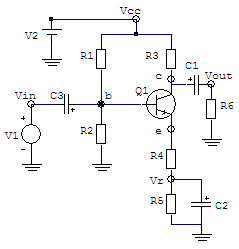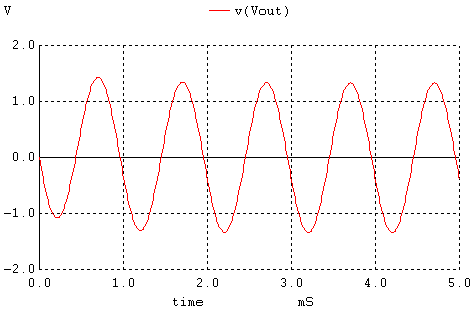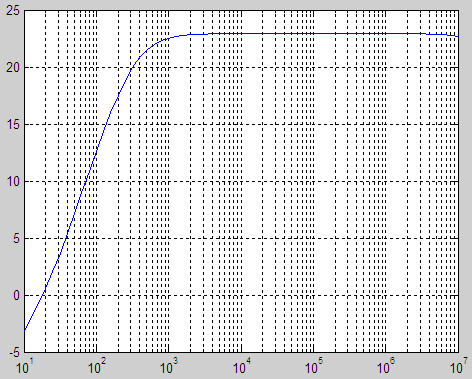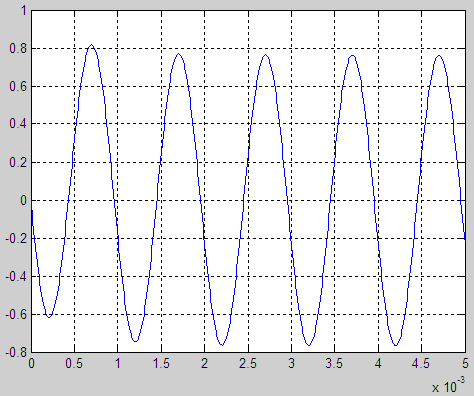WinSpice and Matlab: automation of electronic simulations

In this article, a Matlab routine to drive the WinSpice circuit simulator is
presented. This method can be easily adapted to simulate and drive any circuit entered in this SPICE version.

The performance of the Matlab driver is illustrated by simulating a typical amplifier circuit using some resistors, capacitors and a transistor. In this example we're using Matlab ver. 7.0.1 and WinSpice3 ver. 1.04.07.

SPICE stands for Simulation Program with Integrated Circuit Emphasis

Interchanging information between Matlab and WinSpice allows the implementation of mathematical or optimization algorithms and graphical possibilities not included in the circuit simulator environment itself. The approach developed here is very flexible and utilizes the inherent capability of this SPICE simulator to execute text files and print data to external .csv (comma separated values) files.

First, the circuit in Spice is defined (.cir file). This circuit is simulated and tested within the WinSpice environment before any attempt is made to interact with Matlab.

Second, we prepare the output of the simulator by writing necessary data to an external file, that is, we include 'write' commands in the .cir file, so that the results are saved as external (.csv) text files available to Matlab.

Third, we now generate a new version of the .cir file using Matlab capabilities for manipulating cell-arrays (string matrices), we then insert a Matlab statement to run the Spice simulator from the command line with the .cir filename as a parameter.

After the simulation, we can read the results in the .csv files, process them and act accordingly.

First Step:

We create, test and simulate this circuit in WinSpice:We use these values:

R1 = 10k ohms, R2 = 5k ohms, R3 = 1k ohm,
R4 = 50 ohms, R5 = 1k ohm, R6 = 3k ohms

C1 = C2 = C3 = 10 uF

Q1 = transistor 2N2222

V1(ac) = 0.1V amplitude @ 1k Hz
V2(dc) = 30 V

The .cir file (text file) needed is:

--- Simple amplifier with Transistor ----

V1  Vin  0   dc 0   ac 1   sin(0 0.1 1e3)
V2  Vcc  0   dc 30

R1  Vcc  b   10e3
R2  b    0   5e3
R3  Vcc  c   1ek
R4  e    Vr  50
R5  Vr   0   1e3
R6  Vout 0   3e3

C1  c    Vout  10e-6
C2  Vr   0     10e-6
C3  b    Vin   10e-6

Q1  c    b     e     Q2N2222

.MODEL Q2N2222 NPN
+(IS=3.108E-15 XTI=3 EG=1.11 VAF=131.5 BF=217.5
+ NE=1.541 ISE=190.7E-15 IKF=1.296 XTB=1.5 BR=6.18
+ NC=2 ISC=0 IKR=0 RC=1 CJC=14.57E-12 VJC=.75
+ MJC=.3333 FC=.5 CJE=26.08E-12 VJE=.75 MJE=.3333
+ TR=51.35E-9 TF=451E-12 ITF=.1 VTF=10 XTF=2)

.control
AC DEC 10 10 10MEGHZ
plot vdb(Vout)

TRAN 10E-6 5E-3
plot v(Vout)
.endc
.end

The AC and Transient analysis deliver these results:Second Step:

We include appropriate 'write' commands in the .cir file, so that the results are saved as .csv files available to Matlab.

The control block changes into:

.control
AC DEC 10 10 10MEGHZ
write f_ac_out.csv vdb(Vout)

TRAN 10E-6 5E-3
write f_tran_out.csv v(Vout)
quit
.endc
.end

Third Step:

We now create a function (to be run by Matlab) that can reproduce the above .cir file (simulation of the amplifier), modify relevant parameters with the command 'num2str' (which changes numbers into strings), and launch the circuit simulator. This is the full code that accomplishes it:

function [Rac Rtran] = spice_amp(x)
% Create the file .cir to be run by WinSpice3.
% Built-in function 'num2str' is used to modify parameters
% wherever it is convenient.

b{1} = ['-------------Simple amplifier with Transistor----------- '];
b{2} = [
' '];
b{3} = [
' '];
b{4} = [
'V1  Vin  0   dc 0   ac 1   sin(0 0.1 1e3) '];
b{5} = [
'V2  Vcc  0   dc 30 '];
b{6} = [
' '];
b{7} = [
'R1  Vcc  b   10e3 '];
b{8} = [
'R2  b    0   5e3 '];
b{9} = [
'R3  Vcc  c   ' num2str(x(1))];
b{10} = [
'R4  e    Vr ' num2str(x(2))];
b{11} = [
'R5  Vr   0   1e3 '];
b{12} = [
'R6  Vout 0   3e3 '];
b{13} = [
' '];
b{14} = [
'C1  c    Vout  10e-6 '];
b{15} = [
'C2  Vr   0     10e-6 '];
b{16} = [
'C3  b    Vin   10e-6 '];
b{17} = [
' '];
b{18} = [
'Q1  c    b     e     Q2N2222 '];
b{19} = [
' '];
b{20} = [
'.MODEL Q2N2222 NPN '];
b{21} = [
'+(IS=3.108E-15 XTI=3 EG=1.11 VAF=131.5 BF=217.5 '];
b{22} = [
'+ NE=1.541 ISE=190.7E-15 IKF=1.296 XTB=1.5 BR=6.18 '];
b{23} = [
'+ NC=2 ISC=0 IKR=0 RC=1 CJC=14.57E-12 VJC=.75 '];
b{24} = [
'+ MJC=.3333 FC=.5 CJE=26.08E-12 VJE=.75 MJE=.3333 '];
b{25} = [
'+ TR=51.35E-9 TF=451E-12 ITF=.1 VTF=10 XTF=2) '];
b{26} = [
' '];
b{27} = [
'.control '];
b{28} = [
'AC DEC 10 10 10MEGHZ '];
b{29} = [
'write amp_ac_out.csv vdb(Vout) '];
b{30} = [
' '];
b{31} = [
'TRAN 10E-6 5E-3 '];
b{32} = [
'write amp_tran_out.csv v(Vout) '];
b{33} = [
'quit '];
b{34} = [
'.endc '];
b{35} = [
'.end '];
b{36} = [
' '];

% Save file .cir line-by-line
[r,c] = size(b);
fp = fopen(
'amplifier.cir', 'w+');
for i = 1 : c
fprintf(fp,
'%s\n', b{i});
end
fclose(fp);

% Run WinSpice
% Make sure wspice3.exe and .cir files are available in this directory

! wspice3 amplifier.cir

% Read data saved in .csv files
% (assuming name termination _ac_out.csv and _tran_out.csv)

[Rac Rtran] = getWSpicedata('amp');

This is an important portion of additional code to read the .csv files.

function [Rac Rtran] = getWSpicedata(WSpiceBaseFile)
% Read csv files generated by a 'write' in a 'WSpiceBaseFile' (.cir file)
% Rac{1} = Frequency Points
% Rac{2} = AC response
% Rtran{1} = Time Points
% Rtran{2} = Tran response

ac = fopen([WSpiceBaseFile '_ac_out.csv']);
Rac = textscan(ac,
'%f %*f %f %*f', 'delimiter',',','headerLines', 1);

tran = fopen([WSpiceBaseFile '_tran_out.csv']);
Rtran = textscan(tran,

fclose('all');

Now, we're ready to perform some simulations driving WinSpice from Matlab.

clear; clc; close all

% We try some initial resistors
R3 = 1e3;
R4 = 50;
x = [R3 R4];

% Matlab launches the simulator including our parameters
[Ra Rt] = spice_amplifier(x);

figure
semilogx(Ra{1},Ra{2})
grid
on
figure
plot(Rt{1},Rt{2})
grid
on

% We try another gain modifying one of the resistors
R3 = 500;
x = [R3 R4];
[Ra Rt] = spice_amplifier(x);

figure
semilogx(Ra{1},Ra{2})
grid
on
figure
plot(Rt{1},Rt{2})
grid
on

For R3 = 1k and R4 = 50, the obtained results are:For R3 = 500 and R4 = 50, the obtained results are:We can now use WinSpice as if it was another built-in Matlab function!
This means that we can work through circuit optimization using Matlab...

We can try some numbers in our design, we can see the results and use Matlab to modify relevant values in the circuit, in iterations.

Very powerful
, isn't it?

Reference: